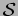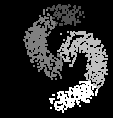## Unsupervised classification by OPF

In this version, as in the supervised one, the samples are nodes of a graph, whose arcs are defined by an adjacency relation between them. The arcs are weighted by the distances between the feature vectors of their corresponding samples and the nodes are also weighted by their probability density values, which are computed from the arc weights.

Letbe a set of relevant maxima in the pdf (e.g., samplesandin Figure 1a). We wish that each sample in the dataset (e.g., samplein Figure 1a) be reached by a path fromwhose minimum density value along it is maximum. The connectivity function assigns to any path in the graph, the minimum between the density values along it and a handicap value of its starting node. The handicap values work as filtering parameters on the pdf, reducing the numbers of clusters by choosing athe relevant maxima. The maximization of the connectivity function for each sample, irrespective to its starting node, partitions the graph into an optimum-path forest, where each root (maximum of the pdf) defines an optimum-path tree (cluster) composed by its most strongly connected samples (Figure 1c).

Figure 1: (a) A pdf of two relevant clusters in a 2D feature space (brighter samples show higher density values). The maximaandcompete for sampleby offering it paths with some strength of connectedness. (b) The influence zones of the pdf's maxima and (c) the influence zones of its relevant maxima.(a) (b) (c)

Some pdf-based approaches assume either explicitly, or often implicitly, that the domes have known shapes and/or can be fitted to parametric functions [9,10,11,12]. Given that the shapes may be far from hyperelliptical, which is the classical assumption, several other methods aim to obtain clusters by avoiding those assumptions [13,14]. Among these approaches, the mean-shift algorithm seems to be the most popular and actively pursued in computer vision [13,15,16,17,18,19,20]. For each sample, it follows the direction of the pdf's gradient vector towards the steepest maximum around that sample. The pdf is never explicitly computed and each maximum should define an influence zone composed by all samples that achieve it. It is not difficult to see that this approach may present problems if the gradient vector is poorly estimated or has magnitude zero. Besides, if a maximum consists of neighboring points with the same density value, it may break its influence zone into multiple ones. This further increases the number of clusters which is usually higher than the desired one.

The unsupervised OPF circumvents those problems by first identifying one sample for each relevant maximum of the pdf and then by defining the influence zone of that maximum (robustness). The connectivity function we use in the feature space is dual of the one used for the IFT-watershed transform from a gray-scale marker in the image domain [21,22], which computes a morphological reconstruction  and a watershed transform  in a same operation. That is, the obtained clusters are equivalent to the dual-watershed regions of the filtered pdf (the pdf without the irrelevant domes), being a more general solution than the one obtained by the popular mean-shift algorithm .